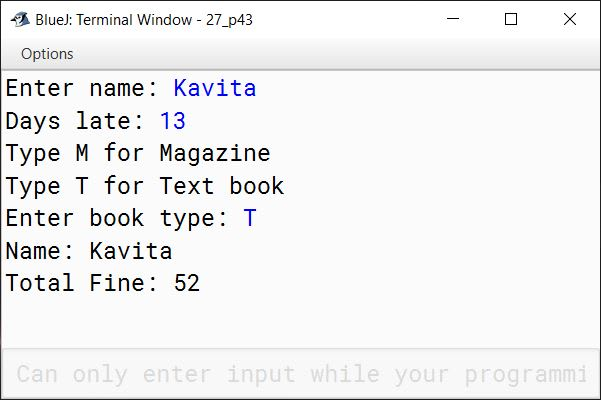# Conditional Constructs in Java

## Multiple Choice Questions

#### Question 1

Which of the following are conditional constructs?

1. if-else
3. switch statement
4. All of these ✓

#### Question 2

Which operator cannot be used with if-else statement?

1. <=
2. ||
3. &&
4. ? : ✓

#### Question 3

What will be the output of the following code?

int size = 2;
if (size < 0)
System.out.println("Small");
else if (size == 0)
System.out.println("Medium");
else
System.out.printIn("Large");
1. Small
2. Large ✓
3. Medium
4. Runtime error

#### Question 4

What will be the output of the following code?

int fruit = 3;
switch (fruit + 1)
{
case 1:
System.out.println("Banana");
break ;
case 2:
System.out.println("Apple");
break ;
case 3:
System.out.println("Orange");
break ;
default :
System.out.println("Fruitless");
}
1. Orange
2. Banana
3. Apple
4. Fruitless ✓

#### Question 5

Predict the output of the following code snippet:

int a = 1;
int b = 2;
if (a == b)
System.out.println ("Both values are equal");
else
System.out.println ("Values are not equal");
1. Both values are equal
2. Incorrect use of the == operator
3. Values are not equal ✓
4. No output

#### Question 6

Consider the following code snippet:

if ( c > d)
x = c;
else
x = d;

Choose the correct option if the code mentioned above is rewritten using the ternary operator:

1. x = (c >d) ? c : d; ✓
2. x = (c >d) ? d : c;
3. x = (c >d) ? c : c;
4. x = (c >d) ? d : d;

#### Question 7

if ((a > b) && (a > c)), then which of the following statements is true?

1. a is the largest number. ✓
2. b is the largest number.
3. c is the largest number.
4. b is the smallest number.

#### Question 8

Consider the following code snippet:

int val = 2;
switch (val)
{
case 1: System.out.println("Case 1");
break;
case 2: System.out.println("Case 2");
break;
default: System.out.println("No match found");
break;
}

Which of the following statements is correct?

1. case 1 will be executed.
2. case 2 will be executed. ✓
3. default will be executed.
4. both case 1 and 2 will be executed.

#### Question 9

A sequence of statements enclosed between a pair of curly brackets is called

1. a compound statement ✓
2. an empty statement
3. a null statement
4. a void statement

#### Question 10

Which clause is optional in the switch statement?

1. default ✓
2. case
3. switch
4. None of these

#### Question 11

Which of the following statements involves a fall-through?

1. if-else
2. for loop
4. switch ✓

#### Question 12

Which of the following causes a fall-through in the switch statement?

1. the omission of fall
2. the omission of continue
3. the omission of break ✓
4. the omission of loop

#### Question 13

Which of the following is mandatory in the switch statement?

1. break
2. continue
3. case ✓
4. default

#### Question 14

Which of the following statement is a valid combination?

1. if inside switch ✓
2. switch inside if ✓
3. else inside switch
4. default inside if

## Assignment Questions

#### Question 1

What is sequential flow of control? Explain with an example.

In sequential flow of control, the statements of a program are executed from top to bottom in order in which they are written including method calls. During execution, the control is transferred to the method called. The method is then executed in a sequential order and upon its completion, the control is transferred back to the calling method and execution of statements continues sequentially. For example, the following program will be executed in a sequential manner:

public class SequentialExample {
static void showGreeting() {
System.out.println("Welcome to KnowledgeBoat");
}

public static void main(String args[]) {
System.out.println("Sequential Execution Program Example");
showGreeting();
}
}

#### Question 2

What is conditional flow of control? Explain with an example.

By default, the statements of a program are executed sequentially from top to bottom in order in which they are written. But most of the times our programs require to alter this top to bottom control flow based on some condition. When the flow of control of a program is changed based on some condition using control statements, it is termed conditional flow of control. For example, in the below program we achieve conditional flow of control using the if-else statement.

public class ConditionalExample {
public static void main(String args[]) {
int x = -10;
if (x >= 0) {
System.out.println("Positive Number");
}
else {
System.out.println("Negative Number");
}
}
}

#### Question 3

Explain the significance of break statement in the switch statement.

The break statement is used inside the switch statement to terminate a statement block. It brings the program control out of the switch statement.

#### Question 4

What is a fall through? Give an example.

break statement at the end of case is optional. Omitting break leads to program execution continuing into the next case and onwards till a break statement is encountered or end of switch is reached. This is termed as fall through. For example, consider the below program:

public class FallThroughExample {
public static void main(String args[]) {
int number = 1;
switch(number) {
case 0:
System.out.println("Value of number is zero");
case 1:
System.out.println("Value of number is one");
case 2:
System.out.println("Value of number is two");
default:
System.out.println("Value of number is greater than two");
}
System.out.println("End of switch");
}
}

Its output when executed will be:

Value of number is one
Value of number is two
Value of number is greater than two
End of switch


#### Question 5

Explain the significance of the default label in the switch statement.

When none of the case values are equal to the expression of switch statement then default case is executed. In the example below, value of number is 4 so case 0, case 1 and case 2 are not equal to number. Hence println statement of default case will get executed printing "Value of number is greater than two" to the console.

int number = 4;
switch(number) {
case 0:
System.out.println("Value of number is zero");
break;
case 1:
System.out.println("Value of number is one");
break;
case 2:
System.out.println("Value of number is two");
break;
default:
System.out.println("Value of number is greater than two");
break;
}
##### Output
Value of number is greater than two


#### Question 6

Explain the use of System.exit(n) method in Java.

The currently running program can be terminated with the help of the exit() method of the System class - System.exit(n). The argument n serves as a status code. A non-zero status code indicates abnormal termination, and a zero status code indicates a normal termination.

#### Question 7

Format the following if statements with indentation:

i. if (x == y) if (x == z) x = 1; else y = 1; else z = 1;

if (x == y)
if (x == z)
x = 1;
else
y = 1;
else
z = 1;

ii. if (x == y) {if (y == z) x = 1; y = 2; } else z = 1;

if (x == y)
{
if (y == z)
x = 1;
y = 2;
}
else
z = 1;

iii. if (num1 != num2) {
if (num2 >= num3) x = 1; y = 2; }
else {x = 1; if (num1 == num2) z = 3;}

if (num1 != num2)
{
if (num2 >= num3)
x = 1;
y = 2;
}
else
{
x = 1;
if (num1 == num2)
z = 3;
}

#### Question 8

Rewrite the following if statement, using the switch statement:

if (choice == 1)
System.out.println("You selected One");
else if (choice == 2)
System.out.println("You selected Two");
else if (choice == 3)
System.out.println("You selected Three");
else if (choice == 4)
System.out.println("You selected Four");
else if (choice == 5)
System.out.println("You selected Five");
else if (choice == 6)
System.out.println("You selected Six");
else
System.out.println("Invalid choice");

switch (choice) {
case 1:
System.out.println("You selected One");
break;

case 2:
System.out.println("You selected Two");
break;

case 3:
System.out.println("You selected Three");
break;

case 4:
System.out.println("You selected Four");
break;

case 5:
System.out.println("You selected Five");
break;

case 6:
System.out.println("You selected Six");
break;

default:
System.out.println("Invalid choice");
}

#### Question 9

Write the following switch statement by using nested if statements:

switch (choice)
{
case 0:
case 1:
x = 111;
y = 222;
break;
case 2:
x = 333;
y = 444;
break;
case 3:
x = -11;
y = -22;
break;
default:
y = 555;
}

if (choice == 0 || choice == 1)
{
x = 111;
y = 222;
}
else
{
if (choice == 2)
{
x = 333;
y = 444;
}
else
{
if (choice == 3)
{
x = -11;
y = -22;
}
else
{
y = 555;
}
}
}

#### Question 10

Write an if statement to find the smallest of the three given integers using the min() method of the Math class.

if (a < Math.min(b, c))
System.out.println(a);
else
System.out.println(Math.min(b, c));

#### Question 11

Find the error in the given statement:

int x = (a => b) ? "a" : "b";

1. => is an invalid operator. it should be >=
2. Type of x should be String.
##### Corrected Statement
String x = (a >= b) ? "a" : "b";

#### Question 12

Find the error, if any, in the following code. Write the correct statement.

int a=5, b=10;
int x = (a>b)>true:false;

1. Ternary operator is written incorrectly.
2. Type of x should be boolean.
##### Corrected Statement
int a=5, b=10;
boolean x = (a>b) ? true:false;

#### Question 13

Rewrite the following statement using if else:

int max=215, min=323;
String str= (max>min) ? "Max is greater than Min" : "Min is Greater than Max";

int max=215, min=323;
String str="";
if (max > min)
str = "Max is greater than Min";
else
str = "Min is Greater than Max";

#### Question 14

What will be the value of 'n' after the execution of the code given below?

int x=2, m=1, c=-1;
int n = x + c;
n = n - c + x;
System.out.println(n);

n will be 4 after execution of the code.

First n = x + c is executed ⇒ n = 2 + (-1) = 1.
Next, n = n - c + x is executed.
n = n - c + x
⇒ n = 1 - (-1) + 2
⇒ n = 4
So final value of n is 4.

#### Question 15

What will be the output of the following code?

int x=2,y=5,a=0;
a=x;
x=y;
y=a;
System.out.println("x=" + x + " y=" + y);

Output is:
x=5 y=2

This code is swapping the values of x and y.

#### Question 16

Find the errors in the following code and rewrite the correct version:

char m="A";
Switch ("A");
{
Case 'a';
System.out.println("A");
break;
Case 'b';
System.out.println("B");
break;
Default:
System.out.println("Not a valid option");
}

The code has the following errors:

1. char variable m is assigned a String literal.
2. S in Switch is capital.
3. There should be no semicolon (;) after switch statement.
4. Expression of switch statement is a String literal "A".
5. C in Case is capital.
6. Semicolon (;) of case statements should be replaced with colon (:).
7. D in Default is capital.

Corrected Version:

char m = 'A';
switch (m) {
case 'a':
System.out.println("A");
break;
case 'b':
System.out.println("B");
break;
default:
System.out.println("Not a valid option");
}

#### Question 17

Write a program to find the number of and sum of all integers greater than 500 and less than 1000 that are divisible by 17

public class KboatDivisibleBy17
{
public static void main(String args[]) {
int sum = 0, count = 0;
for (int i = 501; i < 1000; i++) {
if (i % 17 == 0) {
count++;
sum += i;
}
}
System.out.println("Sum = " + sum);
System.out.println("Count = " + count);
}
}
##### Output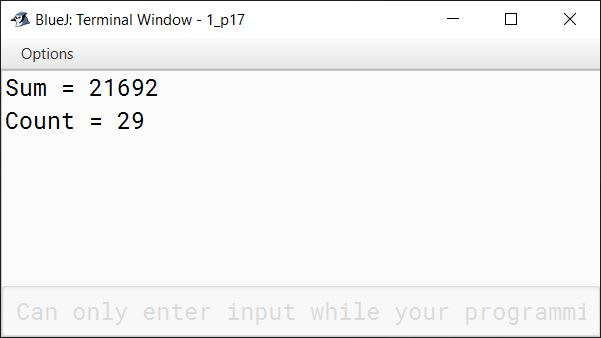#### Question 18

Create a program to find out if the number entered by the user is a two, three or four digits number.

Sample input: 1023
Sample output: 1023 is a 4 digit number.

import java.util.Scanner;

public class KboatNumber
{
public static void main(String args[]) {
Scanner in = new Scanner(System.in);
System.out.print("Enter number: ");
int n = in.nextInt();

if (n >= 10 && n <= 99)
System.out.println("Two Digit Number");
else if (n >= 100 && n <= 999)
System.out.println("Three Digit Number");
else if (n >= 1000 && n <= 9999)
System.out.println("Four Digit Number");
else
System.out.println("Please enter a 2, 3 or 4 digit number");
}
}
##### Output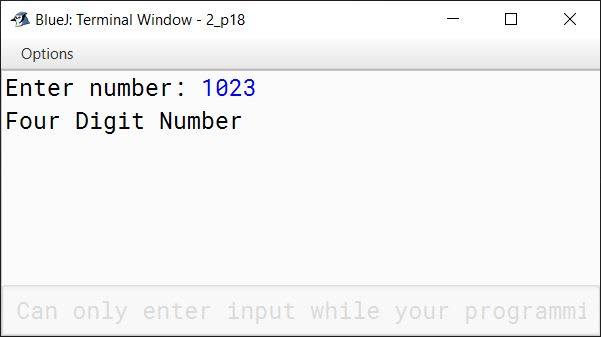#### Question 19

Write a program in Java that reads a word and checks whether it begins with a vowel or not.

import java.util.Scanner;

public class KboatWord
{
public static void main(String args[]) {
Scanner in = new Scanner(System.in);
System.out.print("Enter a word: ");
String w = in.nextLine();
char ch = w.charAt(0);
ch = Character.toUpperCase(ch);
if (ch == 'A' ||
ch == 'E' ||
ch == 'I' ||
ch == 'O' ||
ch == 'U')
System.out.println("Word begins with a vowel");
else
System.out.println("Word does not begin with a vowel");
}
}
##### Output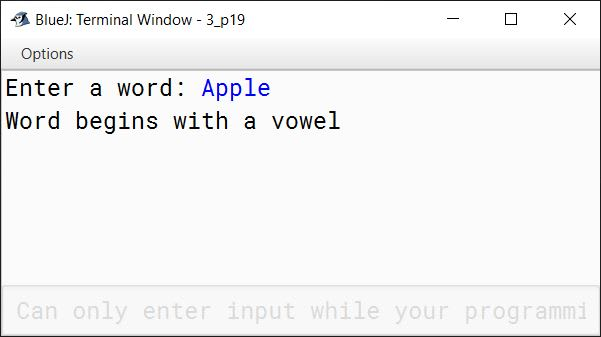#### Question 20

Write a program in Java to read three integers and display them in descending order.

import java.util.Scanner;

public class KboatDescending
{
public static void main(String args[]) {
Scanner in = new Scanner(System.in);
System.out.print("Enter first number: ");
int a = in.nextInt();
System.out.print("Enter second number: ");
int b = in.nextInt();
System.out.print("Enter third number: ");
int c = in.nextInt();

int max = -1, mid = -1, min = -1;
if (a > b) {
if (a > c) {
max = a;
if (b > c) {
mid = b;
min = c;
}
else {
mid = c;
min = b;
}
}
else {
max = c;
mid = a;
min = b;
}
}
else {
if (b > c) {
max = b;
if (a > c) {
mid = a;
min = c;
}
else {
{
mid = c;
min = a;
}
}
}
else {
max = c;
mid = b;
min = a;
}
}

System.out.println("Numbers in Descending order:");
System.out.println(max + " " + mid + " " + min);
}
}
##### Output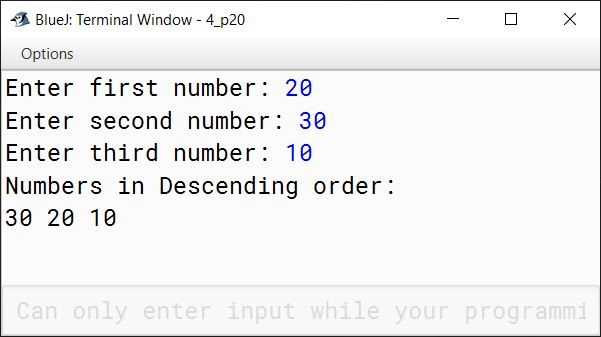#### Question 21

Using the ternary operator, create a program to find the largest of three numbers.

import java.util.Scanner;

public class KboatLargestNumber
{
public static void main(String args[]) {
Scanner in = new Scanner(System.in);
System.out.print("Enter first number: ");
int a = in.nextInt();
System.out.print("Enter second number: ");
int b = in.nextInt();
System.out.print("Enter third number: ");
int c = in.nextInt();

int max = a > b ? a : b;
max = max > c ? max : c;

System.out.print("Largest Number = " + max);
}
}
##### Output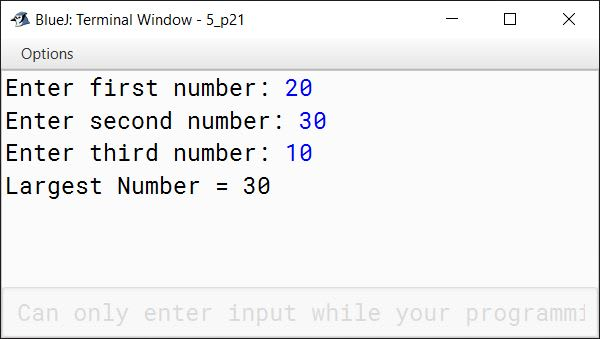#### Question 22

Write a program that reads a month number and displays it in words.

import java.util.Scanner;

public class KboatMonthInWords
{
public static void main(String args[]) {
Scanner in = new Scanner(System.in);
System.out.print("Enter month number: ");
int n = in.nextInt();
switch(n) {
case 1:
System.out.println("January");
break;

case 2:
System.out.println("Feburary");
break;

case 3:
System.out.println("March");
break;

case 4:
System.out.println("April");
break;

case 5:
System.out.println("May");
break;

case 6:
System.out.println("June");
break;

case 7:
System.out.println("July");
break;

case 8:
System.out.println("August");
break;

case 9:
System.out.println("September");
break;

case 10:
System.out.println("October");
break;

case 11:
System.out.println("November");
break;

case 12:
System.out.println("December");
break;

default:
System.out.println("Wrong month number");
break;
}
}
}
##### Output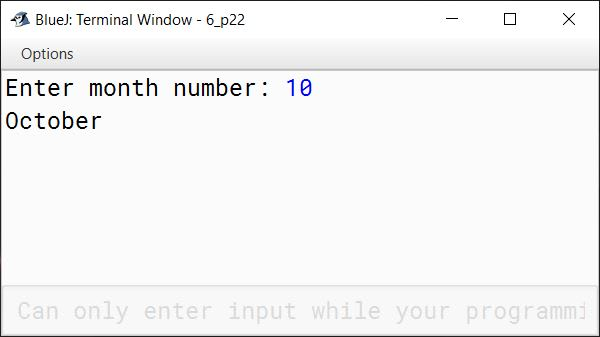#### Question 23

Admission in a professional course is subject to the following criteria:

1. Marks in Physics >= 70
2. Marks in Chemistry >= 60
3. Marks in Mathematics >= 70
4. Total marks in all subjects >= 225
Or
Total marks in Physics and Mathematics >= 150

Write a program in Java to accept marks in these 3 subjects (Physics, Chemistry, and Mathematics) and display if a candidate is eligible.

import java.util.Scanner;

{
public static void main(String args[]) {
Scanner in = new Scanner(System.in);
System.out.print("Enter marks in Physics: ");
int p = in.nextInt();
System.out.print("Enter marks in Chemistry: ");
int c = in.nextInt();
System.out.print("Enter marks in Mathematics: ");
int m = in.nextInt();
int t = p + c + m;
int pm = p + m;

if (p >= 70 && c >= 60 && m >= 70
&& (t >= 225 || pm >= 150))
System.out.println("Eligible");
else
System.out.println("Not Eligible");
}
}
##### Output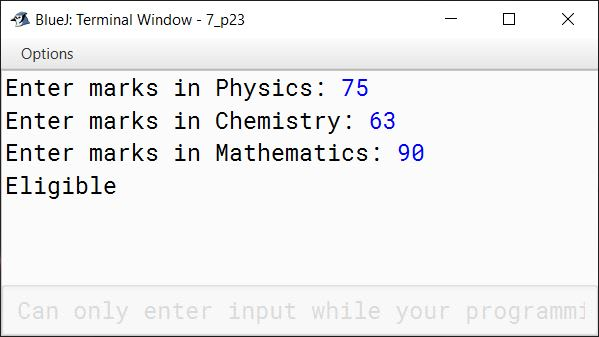#### Question 24

Using the switch statement in Java, write a program to display the name of the city according to the user's choice.
D — Delhi, M — Mumbai, K — Kolkata, C — Chennai

import java.util.Scanner;

public class KboatCityName
{
public static void main(String args[]) {
Scanner in = new Scanner(System.in);
char ch = in.next().charAt(0);
switch (ch) {
case 'D':
System.out.println("Delhi");
break;

case 'M':
System.out.println("Mumbai");
break;

case 'K':
System.out.println("Kolkata");
break;

case 'C':
System.out.println("Chennai");
break;

default:
System.out.println("Invalid choice");
}
}
}
##### Output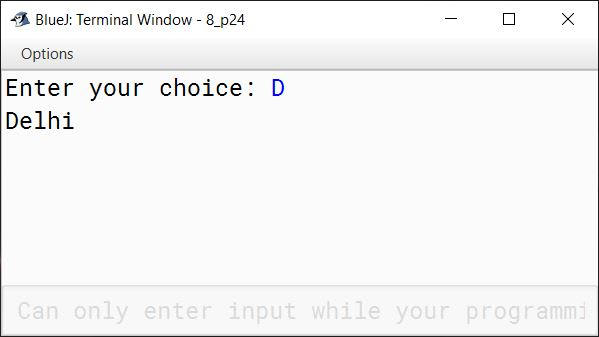#### Question 25

Write a program that will read the value of x and compute the following function:

$y = \begin{cases} 7 &\text{for } \text{x } \text{\textgreater} \text{ 0} \\ 0 &\text{for } x = 0 \\ -7 &\text{for } \text{x } \text{\textless} \text{ 0} \end{cases}$

import java.util.Scanner;

public class KboatFunction
{
public static void main(String args[]) {
Scanner in = new Scanner(System.in);
System.out.print("Enter x: ");
int x = in.nextInt();
int y = -1;
if (x > 0)
y = 7;
else if (x == 0)
y = 0;
else
y = -7;

System.out.println("y=" + y);
}
}
##### Output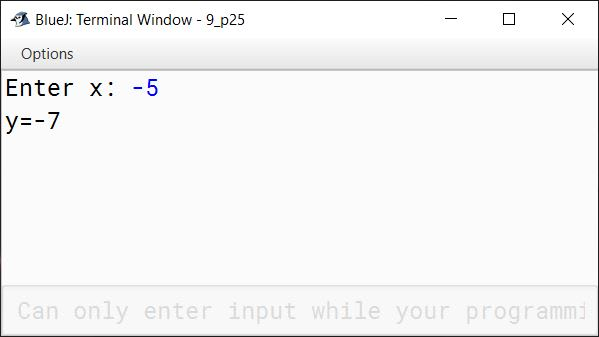#### Question 26

Employees at Arkenstone Consulting earn the basic hourly wage of Rs.500. In addition to this, they also receive a commission on the sales they generate while tending the counter. The commission given to them is calculated according to the following table:

Total SalesCommmision Rate
Rs. 100 to less than Rs. 10001%
Rs. 1000 to less than Rs. 100002%
Rs. 10000 to less than Rs. 250003%
Rs. 25000 and above3.5%

Write a program in Java that inputs the number of hours worked and the total sales. Compute the wages of the employees.

import java.util.Scanner;

public class KboatArkenstoneConsulting
{
public static void main(String args[]) {
Scanner in = new Scanner(System.in);
System.out.print("Enter number of hours: ");
int hrs = in.nextInt();
System.out.print("Enter total sales: ");
int sales = in.nextInt();
double wage = hrs * 500;
double c = 0;
if (sales < 100)
c = 0;
else if (sales < 1000)
c = 1;
else if (sales < 10000)
c = 2;
else if (sales < 25000)
c = 3;
else
c = 3.5;

double comm = c * sales / 100.0;
wage += comm;

System.out.println("Wage = " + wage);
}
}
##### Output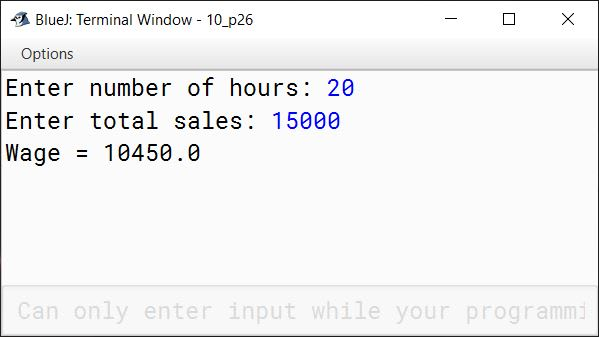#### Question 27

Write a program in Java to compute the perimeter and area of a triangle, with its three sides given as a, b, and c using the following formulas:

$\text{Perimeter} = a + b + c$

$\text{Area} = \sqrt{s(s - a)(s - b)(s - c)}$

$\text{Where} \space s = \dfrac{a+b+c}{2}$

import java.util.Scanner;

public class KboatTriangle
{
public static void main(String args[]) {
Scanner in = new Scanner(System.in);
System.out.print("Enter first side: ");
double a = in.nextDouble();
System.out.print("Enter second side: ");
double b = in.nextDouble();
System.out.print("Enter third side: ");
double c = in.nextDouble();

double perimeter = a + b + c;
double s = perimeter / 2;
double area = Math.sqrt(s * (s - a) * (s - b) * (s - c));

System.out.println("Perimeter = " + perimeter);
System.out.println("Area = " + area);
}
}
##### Output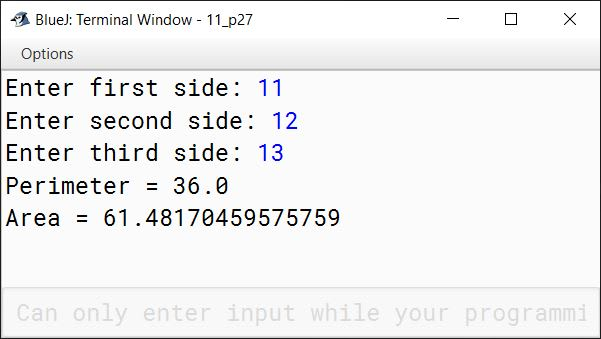#### Question 28

Mayur Transport Company charges for parcels as per the following tariff:

WeightCharges
Upto 10 Kg.Rs. 30 per Kg.
For the next 20 Kg.Rs. 20 per Kg.
Above 30 Kg.Rs. 15 per Kg.

Write a program in Java to calculate the charge for a parcel, taking the weight of the parcel as an input.

import java.util.Scanner;

public class KboatMayurTpt
{
public static void main(String args[]) {
Scanner in = new Scanner(System.in);
System.out.print("Enter parcel weight: ");
double wt = in.nextDouble();
double amt = 0;

if (wt <= 10)
amt = 30 * wt;
else if (wt <= 30)
amt = 300 + ((wt - 10) * 20);
else
amt = 300 + 400 + ((wt - 30) * 15);

System.out.println("Parcel Charge = " + amt);
}
}
##### Output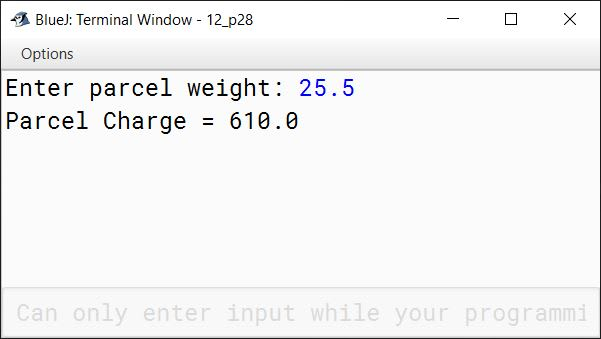#### Question 29

Create a program in Java to find out if a number entered by the user is a Duck Number.

A Duck Number is a number which has zeroes present in it, but there should be no zero present in the beginning of the number. For example, 6710, 8066, 5660303 are all duck numbers whereas 05257, 080009 are not.

import java.io.BufferedReader;
import java.io.IOException;

public class KboatDuckNumber
{
public void duckNumberCheck() throws IOException {

System.out.print("Enter number: ");
boolean isDuck = false;
boolean firstZero = false;
int c = 0, d;

/*
* 10 is the ASCII code of newline
* We will read from inputstream
* one character at a time till we
* encounter a newline i.e. enter
*/
while((d = in.read()) != 10) {

char ch = (char)d;

if (c == 0 && ch == '0'  )
firstZero = true;

if (!firstZero && ch == '0')
isDuck = true;

c++;
}

if (isDuck)
System.out.println("Duck Number");
else
System.out.println("Not a Duck Number");
}
}
##### Output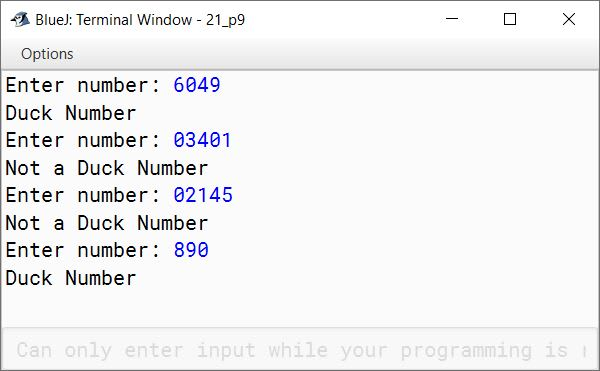#### Question 30

The electricity board charges the bill according to the number of units consumed and the rate as given below:

Units ConsumedRate Per Unit
First 100 units80 Paisa per unit
Next 200 unitsRs. 1 per unit
Above 300 unitsRs. 2.50 per unit

Write a program in Java to accept the total units consumed by a customer and calculate the bill. Assume that a meter rent of Rs. 500 is charged from the customer.

import java.util.Scanner;

public class KboatElectricBill
{
public static void main(String args[]) {
Scanner in = new Scanner(System.in);
System.out.print("Enter Units Consumed: ");
int units = in.nextInt();
double amt = 0.0;
if (units <= 100)
amt = units * 0.8;
else if (units <= 300)
amt = (100 * 0.8) + ((units - 100) * 1);
else
amt = (100 * 0.8) + (200 * 1.0) + ((units - 300) * 2.5);

amt += 500;

System.out.println("Units Consumed: " + units);
System.out.println("Bill Amount: " + amt);
}
}
##### Output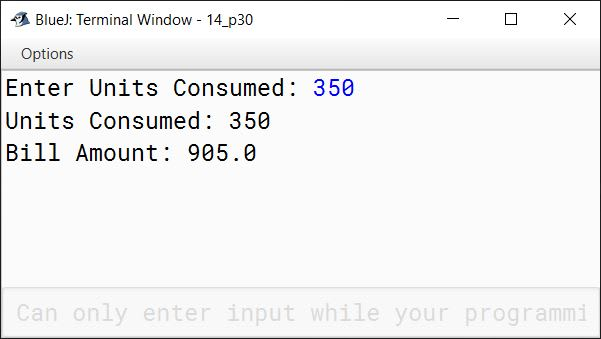#### Question 31

Write a menu driven program to accept a number from the user and check whether it is a Buzz number or an Automorphic number.

i. Automorphic number is a number, whose square's last digit(s) are equal to that number. For example, 25 is an automorphic number, as its square is 625 and 25 is present as the last two digits.
ii. Buzz number is a number, that ends with 7 or is divisible by 7.

import java.util.Scanner;

public class KboatBuzzAutomorphic
{
public static void main(String args[]) {
Scanner in = new Scanner(System.in);
System.out.println("1. Buzz number");
System.out.println("2. Automorphic number");
int choice = in.nextInt();
System.out.print("Enter number: ");
int num = in.nextInt();

switch (choice) {
case 1:
if (num % 10 == 7 || num % 7 == 0)
System.out.println(num + " is a Buzz Number");
else
System.out.println(num + " is not a Buzz Number");
break;

case 2:
int sq = num * num;
int d = 0;
int t = num;

/*
* Count the number of
* digits in num
*/
while(t > 0) {
d++;
t /= 10;
}

/*
* Extract the last d digits
* from square of num
*/
int ld = (int)(sq % Math.pow(10, d));

if (ld == num)
System.out.println(num + " is automorphic");
else
System.out.println(num + " is not automorphic");
break;

default:
System.out.println("Incorrect Choice");
break;
}
}
}
##### Output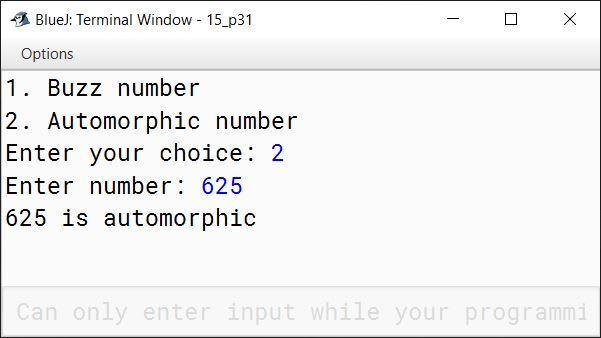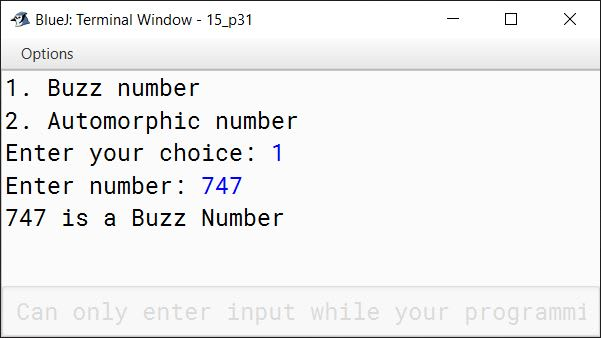#### Question 32

Write a Java program in which you input students name, class, roll number, and marks in 5 subjects. Find out the total marks, percentage, and grade according to the following table.

>=90A+
>=80 and <90A
>=70 and <80B+
> =60 and <70B
>=50 and <60C
>=40 and <50D
<40E

import java.util.Scanner;

{
public static void main(String args[]) {
Scanner in = new Scanner(System.in);
System.out.print("Enter name of student: ");
String n = in.nextLine();
System.out.print("Enter class of student: ");
int c = in.nextInt();
System.out.print("Enter roll no of student: ");
int r = in.nextInt();
System.out.print("Enter marks in 1st subject: ");
int m1 = in.nextInt();
System.out.print("Enter marks in 2nd subject: ");
int m2 = in.nextInt();
System.out.print("Enter marks in 3rd subject: ");
int m3 = in.nextInt();
System.out.print("Enter marks in 4th subject: ");
int m4 = in.nextInt();
System.out.print("Enter marks in 5th subject: ");
int m5 = in.nextInt();
int t = m1 + m2 + m3 + m4 + m5;
double p = t / 500.0 * 100.0;
String g = "";
if (p >= 90)
g = "A+";
else if (p >= 80)
g = "A";
else if (p >=70)
g = "B+";
else if (p >= 60)
g = "B";
else if (p >= 50)
g = "C";
else if (p >= 40)
g = "D";
else
g = "E";

System.out.println("Total Marks = " + t);
System.out.println("Percentage = " + p);
}
}
##### Output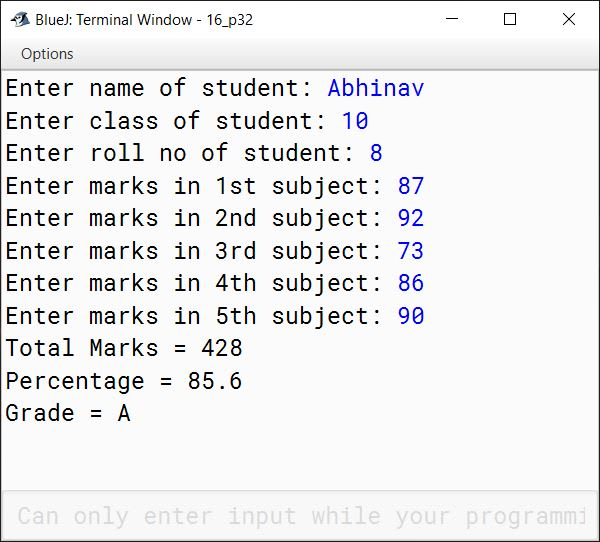#### Question 33

Write a program in Java to accept three numbers and check whether they are Pythagorean Triplet or not. The program must display the message accordingly. [Hint: h2=p2+b2]

import java.util.Scanner;

public class KboatPythagoreanTriplet
{
public static void main(String args[]) {
Scanner in = new Scanner(System.in);
System.out.print("Enter 1st number: ");
int a = in.nextInt();
System.out.print("Enter 2nd number: ");
int b = in.nextInt();
System.out.print("Enter 3rd number: ");
int c = in.nextInt();

if (a * a + b * b == c * c ||
a * a + c * c == b * b ||
b * b + c * c == a * a)
System.out.println("Pythagorean Triplets");
else
System.out.println("Not Pythagorean Triplets");
}
}
##### Output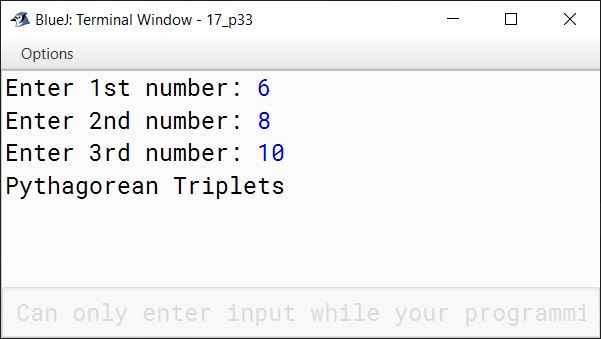#### Question 34

Using switch case, write a program to convert temperature from Fahrenheit to Celsius and Celsius to Fahrenheit.
Fahrenheit to Celsius formula: (32°F - 32) x 5/9
Celsius to Fahrenheit formula: (0°C x 9/5) + 32

import java.util.Scanner;

public class KboatTemperature
{
public static void main(String args[]) {
Scanner in = new Scanner(System.in);
System.out.println("Type 1 for Fahrenheit to Celsius");
System.out.println("Type 2 for Celsius to Fahrenheit");
int choice = in.nextInt();
double ft = 0.0, ct = 0.0;

switch (choice) {
case 1:
System.out.print("Enter temperature in Fahrenheit: ");
ft = in.nextDouble();
ct = (ft - 32) * 5 / 9.0;
System.out.println("Temperature in Celsius: " + ct);
break;

case 2:
System.out.print("Enter temperature in Celsius: ");
ct = in.nextDouble();
ft = 9.0 / 5.0 * ct + 32;
System.out.println("Temperature in Fahrenheit: " + ft);
break;

default:
System.out.println("Incorrect Choice");
break;
}
}
}
##### Output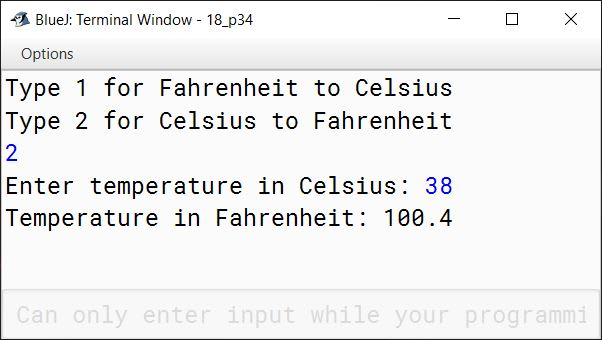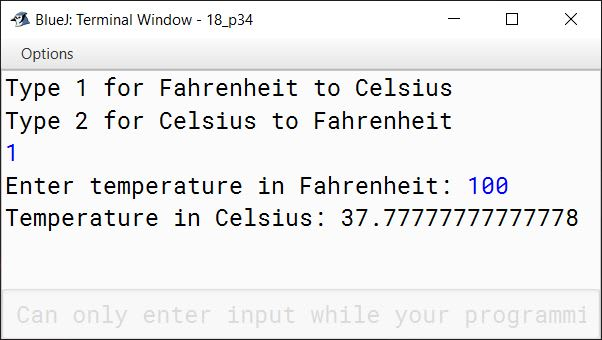#### Question 35

Star mall is offering discount on various types of products purchased by its customers. Following table shows different type of products and their respective code along with the discount offered. Based on the code entered, the mall is calculating the total amount after deducting the availed discount. Create a program to calculate total amount to be paid by the customer.

ItemItem CodeDiscount
LaptopL5%
LCDD7%
XBoxX10%
PrinterP11%

import java.util.Scanner;

public class KboatStarMall
{
public static void main(String args[]) {
Scanner in = new Scanner(System.in);
System.out.print("Enter purchase amount: ");
double amt = in.nextDouble();
System.out.print("Enter item code: ");
char code = in.next().charAt(0);
int d = 0;
switch (code) {
case 'L':
d = 5;
break;

case 'D':
d = 7;
break;

case 'X':
d = 10;
break;

case 'P':
d = 11;
break;

default:
System.out.println("Invalid Item Code");
}

double disc = amt * d / 100.0;
double total = amt - disc;

System.out.println("Total amount payable = " + total);
}
}
##### Output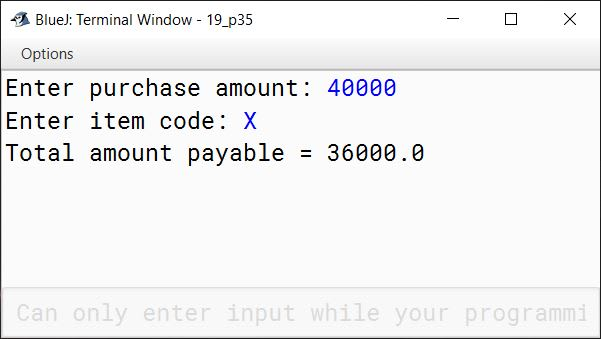#### Question 36

A cloth showroom has announced the following festival discounts on the purchase of items based on the total cost of the items purchased:

Total CostDiscount Rate
Less than Rs. 20005%
Rs. 2000 to less than Rs. 500025%
Rs. 5000 to less than Rs. 10,00035%
Rs. 10,000 and above50%

Write a program to input the total cost and to compute and display the amount to be paid by the customer availing the the discount.

import java.util.Scanner;

public class KboatClothDiscount
{
public static void main(String args[]) {
Scanner in = new Scanner(System.in);
System.out.print("Enter total cost: ");
double cost = in.nextDouble();
double amt;

if (cost < 2000) {
amt = cost - (cost * 5 / 100.0);
}
else if (cost < 5000) {
amt = cost - (cost * 25 / 100.0);
}
else if (cost < 10000) {
amt = cost - (cost * 35 / 100.0);
}
else {
amt = cost - (cost * 50 / 100.0);
}

System.out.println("Amount to be paid: " + amt);

}
}
##### Output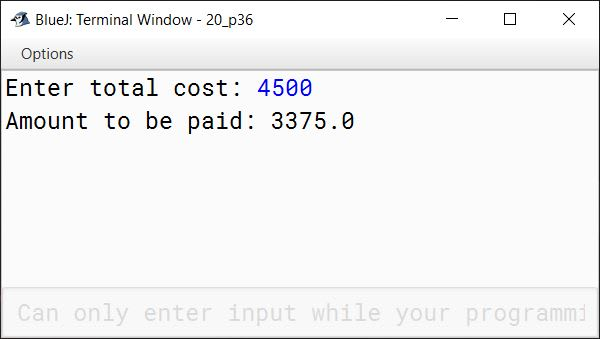#### Question 37

A cloth manufacturing company offers discounts to the dealers and retailers. The discount is computed using the length of the cloth as per the following table:

Length of clothDealer's DiscountRetailer's Discount
Up to 1000 meters20%15%
Above 1000 meters but less than 2000 meters25%20%
More than 2000 meters35%25%

Write a program in Java to input the length of the cloth and the total amount of purchase. The program should display a menu to accept type of customer — Dealer (D) or Retailer (R) and print the amount to be paid. Display a suitable error message for a wrong choice.

import java.util.Scanner;

public class KboatDiscount
{
public static void main(String args[]) {
Scanner in = new Scanner(System.in);
System.out.print("Enter Cloth length: ");
int l = in.nextInt();
System.out.print("Enter Total Amount of purchase: ");
double a = in.nextDouble();
System.out.println("Enter D for Dealer: ");
System.out.println("Enter R for Retailer: ");
System.out.print("Enter customer type: ");
char type = in.next().charAt(0);
type = Character.toUpperCase(type);
int d = 0;
switch (type) {
case 'D':
if (l <= 1000)
d = 20;
else if (l <= 2000)
d = 25;
else
d = 35;
break;

case 'R':
if (l <= 1000)
d = 15;
else if (l <= 2000)
d = 20;
else
d = 25;
break;

default:
System.out.println("Wrong choice");
}

double disc = a * d / 100.0;
double amt = a - disc;

System.out.println("Amount to be paid = " + amt);
}
}
##### Output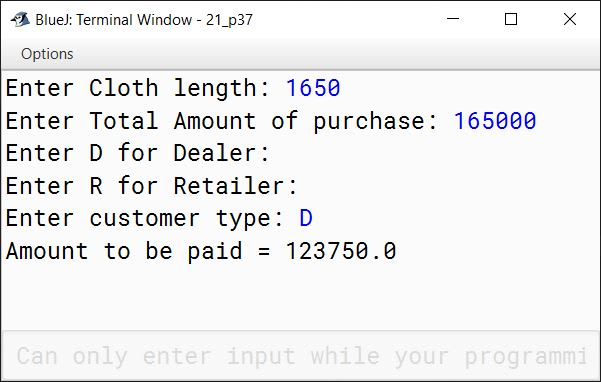#### Question 38

Using switch case statement in Java, create a program to convert rupee into dollar and dollar into rupee according to the user's choice. Assume conversion price: 1 Dollar = Rs.77.

import java.util.Scanner;

public class KboatRupeeDollar
{
public static void main(String args[]) {
Scanner in = new Scanner(System.in);
System.out.println("Type 1 for Rupee to Dollar conversion: ");
System.out.println("Type 2 for Dollar to Rupee conversion: ");
int choice = in.nextInt();
switch (choice) {
case 1:
System.out.print("Enter rupee amount: ");
double r1 = in.nextDouble();
double d1 = r1 / 77;
System.out.println(r1 + " rupees => " + d1 + " dollars");
break;

case 2:
System.out.print("Enter dollar amount: ");
double d2 = in.nextDouble();
double r2 = d2 * 77;
System.out.println(d2 + " dollars => " + r2 + " rupees");
break;

default:
System.out.println("Incorrect Choice");
}
}
}
##### Output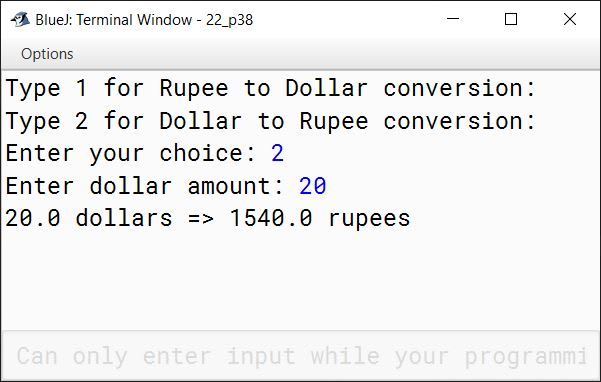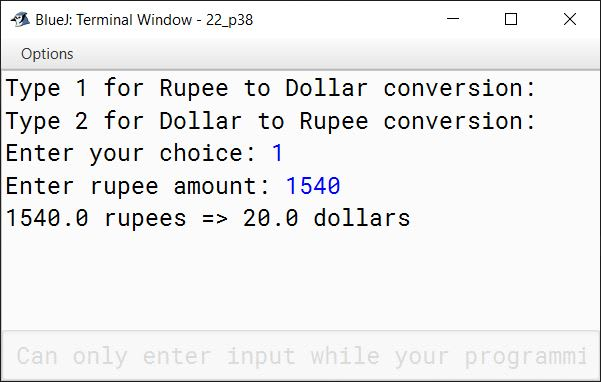#### Question 39

A box of cookies can hold 24 cookies, and a container can hold 75 boxes of cookies. Write a program that prompts the user to enter the total number of cookies, the number of cookies in each box, and the number of cookies boxes in a container. The program then outputs the number of boxes and the number of containers required to ship the cookies.

import java.util.Scanner;

{
public static void main(String args[]) {
Scanner in = new Scanner(System.in);
System.out.print("Enter total no. of cookies: ");
System.out.print("Enter no. of cookies per box: ");
System.out.print("Enter no. of boxes per container: ");
int boxPerContainer = in.nextInt();

numBox++;

int numContainer = numBox / boxPerContainer;
numContainer++;

System.out.println("No. of boxes = " + numBox);
System.out.println("No. of containers = " + numContainer);
}
}
##### Output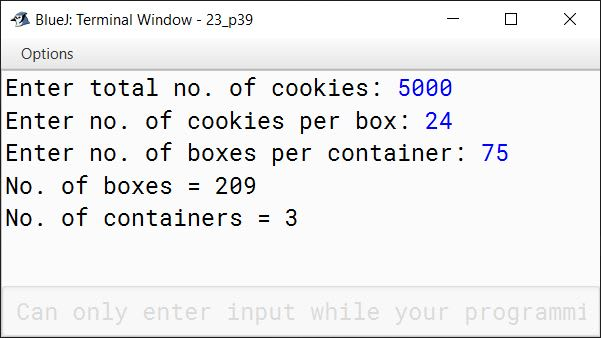#### Question 40

Conversion Table
============

1. Milliseconds to Seconds
2. Milliseconds to Minutes
3. Seconds to Milliseconds
4. Seconds to Minutes
5. Minutes to Milliseconds
6. Minutes to Seconds

For an incorrect choice, display an appropriate error message.

Hint: 1 second = 1000 milliseconds

import java.util.Scanner;

public class KboatTimeConversion
{
public static void main(String args[]) {
Scanner in = new Scanner(System.in);
System.out.println("Conversion Table");
System.out.println("================");
System.out.println("1. Milliseconds to Seconds");
System.out.println("2. Milliseconds to Minutes");
System.out.println("3. Seconds to Milliseconds");
System.out.println("4. Seconds to Minutes");
System.out.println("5. Minutes to Milliseconds");
System.out.println("6. Minutes to Seconds");
int choice = in.nextInt();
double ip = 0, op = 0;
switch (choice) {
case 1:
System.out.print("Enter Milliseconds: ");
ip = in.nextDouble();
op = ip / 1000;
System.out.println("Seconds = " + op);
break;

case 2:
System.out.print("Enter Milliseconds: ");
ip = in.nextDouble();
op = ip / 1000 / 60;
System.out.println("Minutes = " + op);
break;

case 3:
System.out.print("Enter Seconds: ");
ip = in.nextDouble();
op = ip * 1000;
System.out.println("Milliseconds = " + op);
break;

case 4:
System.out.print("Enter Seconds: ");
ip = in.nextDouble();
op = ip / 60;
System.out.println("Minutes = " + op);
break;

case 5:
System.out.print("Enter Minutes: ");
ip = in.nextDouble();
op = ip * 60 * 1000;
System.out.println("Milliseconds = " + op);
break;

case 6:
System.out.print("Enter Minutes: ");
ip = in.nextDouble();
op = ip * 60;
System.out.println("Seconds = " + op);
break;

default:
System.out.println("Incorrect choice");
}
}
}
##### Output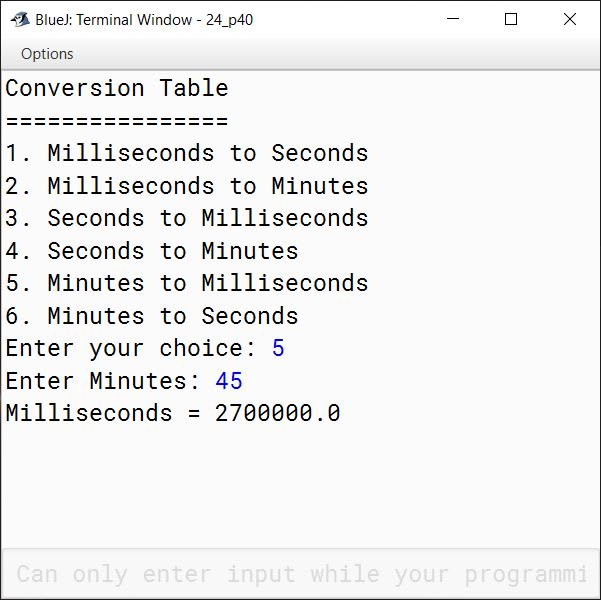#### Question 41

Using the switch statement, write a menu driven program:

1. To check and display whether a number input by the user is a composite number or not.
A number is said to be composite, if it has one or more than one factors excluding 1 and the number itself.
Example: 4, 6, 8, 9...
2. To find the smallest digit of an integer that is input:
Sample input: 6524
Sample output: Smallest digit is 2
For an incorrect choice, an appropriate error message should be displayed.

import java.util.Scanner;

public class KboatNumber
{
public static void main(String args[]) {
Scanner in = new Scanner(System.in);
System.out.println("Type 1 for Composite Number");
System.out.println("Type 2 for Smallest Digit");
int ch = in.nextInt();

switch (ch) {
case 1:
System.out.print("Enter Number: ");
int n = in.nextInt();
int c = 0;
for (int i = 1; i <= n; i++) {
if (n % i == 0)
c++;
}

if (c > 2)
System.out.println("Composite Number");
else
System.out.println("Not a Composite Number");
break;

case 2:
System.out.print("Enter Number: ");
int num = in.nextInt();
int s = 10;
while (num != 0) {
int d = num % 10;
if (d < s)
s = d;
num /= 10;
}
System.out.println("Smallest digit is " + s);
break;

default:
System.out.println("Wrong choice");
}
}
}
##### Output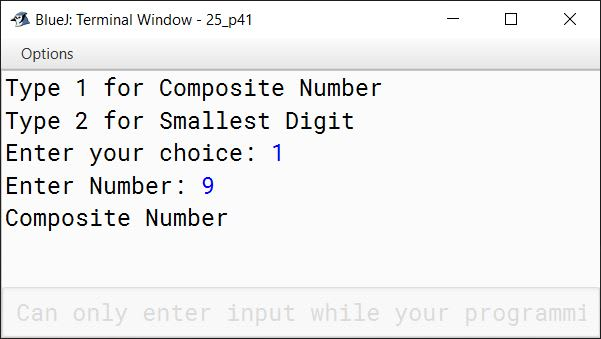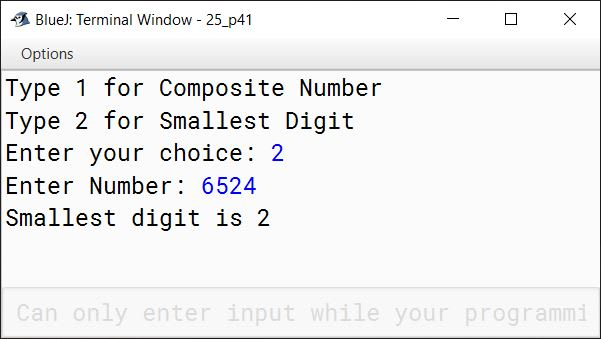#### Question 42

A new taxi service based on electric vehicles is offering services within a metro city. Following is the fare chart including the type of taxi used to commute and price per kilometer. Create a program to calculate total fare depending on the distance travelled in kilometers.

TypeFare
Micro10.05/Km
Macro15.05/Km
Shared7.05/Km

import java.util.Scanner;

public class KboatTaxi
{
public static void main(String args[]) {
Scanner in = new Scanner(System.in);
System.out.println("Type 1 for Micro Taxi");
System.out.println("Type 2 for Macro Taxi");
System.out.println("Type 3 for Shared Taxi");
System.out.print("Enter Taxi Type: ");
int ch = in.nextInt();
System.out.print("Enter distance travelled: ");
int dist = in.nextInt();
double fare = 0;

switch (ch) {
case 1:
fare = 10.05;
break;

case 2:
fare = 15.05;
break;

case 3:
fare = 7.05;
break;

default:
System.out.println("Wrong choice");
}

double totalFare = fare * dist;
System.out.println("Total Fare = " + totalFare);
}
}
##### Output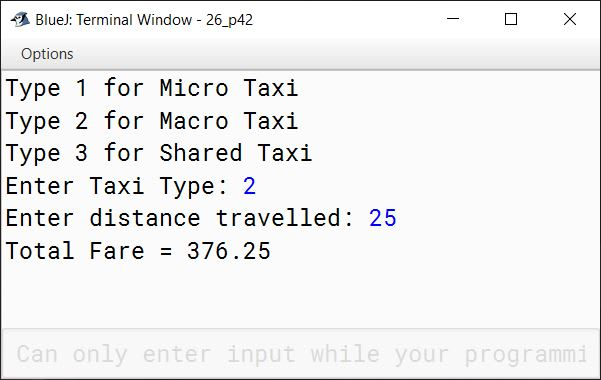#### Question 43

The City Library charges late fine from members if the books were not returned on time as per the following table:

Number of days lateMagazines fine per dayText books fine per day
Up to 5 daysRs. 1Rs. 2
6 to 10 daysRs. 2Rs. 3
11 to 15 daysRs. 3Rs. 4
16 to 20 daysRs. 5Rs. 6
More than 20 daysRs. 6Rs. 7

Using the switch statement, write a program in Java to input name of person, number of days late and type of book — 'M' for Magazine and 'T' for Text book. Compute the total fine and display it along with the name of the person.

import java.util.Scanner;

public class KboatLibrary
{
public static void main(String args[]) {
Scanner in = new Scanner(System.in);
System.out.print("Enter name: ");
String name = in.nextLine();
System.out.print("Days late: ");
int days = in.nextInt();
System.out.println("Type M for Magazine");
System.out.println("Type T for Text book");
System.out.print("Enter book type: ");
char type = in.next().charAt(0);
int fine = 0;
switch (type) {
case 'M':
if (days <= 5)
fine = 1;
else if (days <= 10)
fine = 2;
else if (days <= 15)
fine = 3;
else if (days <= 20)
fine = 5;
else
fine = 6;
break;

case 'T':
if (days <= 5)
fine = 2;
else if (days <= 10)
fine = 3;
else if (days <= 15)
fine = 4;
else if (days <= 20)
fine = 6;
else
fine = 7;
break;

default:
System.out.println("Invalid book type");
}

int totalFine = fine * days;
System.out.println("Name: " + name);
System.out.println("Total Fine: " + totalFine);
}
}
##### Output